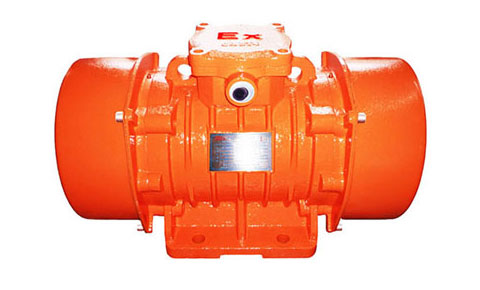# How to Choose the Power of the Vibration Motor?

The power of the vibration motor should be selected according to the power required by the production machinery, and try to make the vibration motor run under the rated load. When choosing, you should pay attention to the following two points:

1. If the power of the vibration motor is selected too small, the long-term overload of the vibration motor will occur. It will be damaged due to heat, and even the vibration motor will be burned.

2. If the power of the vibration motor is selected too large, the output mechanical power of the motor cannot be fully utilized, and the power factor and efficiency are not high.How to choose the power of the vibration motor?

To correctly select the power of the vibration motor, the following calculations or comparisons must be made:

For the continuous working mode with constant load, if you know the power of the load (that is, the power on the production mechanical shaft) PI(kW), you can calculate the required power P(kW) of the vibration motor as follows: P=P1/n1n2, where n1 is the efficiency of the production machinery; n2 is the efficiency of the vibration motor. That is the transmission efficiency.

The power obtained by the above formula is not necessarily the same as the product power. Therefore, the rated power of the selected vibration motor should be equal to or slightly larger than the calculated power.

Example: The power of a production machine is 3.95kw, and the mechanical efficiency is 70%. If a vibration motor with an efficiency of 0.8 is selected, how many kw should the power of the vibration motor be? Solution: P=P1/n1n2=3.95 /0.7*0.8=7.1kw, because there is no 7.1 kW this-specification. So choose 7.5kW vibration motor.

Vibration motor with short-term work quota. Compared with the vibration motor with the same power continuous duty rating. The maximum torque is large, the weight is small, and the price is low. Therefore, when conditions permit, the vibration motor with short-term work quota should be selected as far as possible.

For a vibration motor with a rated intermittent operation, the selection of its power should be based on the size of the load duration rate, and a vibration motor specially used for intermittent operation should be selected. The formula for calculating the load continuous string Fs% is: FS% =tg/(tg+t.)×100%, where tg is the working time, t. is the stop time min ; tgtt. is the working cycle time min.

In addition, the analogy method can also be used to select the power of the vibration motor. The so-called analogy method is to compare the power of the vibration motor used in similar production machinery. The specific method is: to understand the power of the vibration motor used by the similar production machinery of this unit or other nearby units, and then select the vibration motor of similar power to conduct a test run. The purpose of the test run is to verify that the selected vibration motor matches the production machinery.

The verification method is: make it drive the production machinery to run, measure the working current of the vibration motor with a clamp ammeter, and compare the measured current with the rated current marked on the nameplate of the vibration motor. If the actual working current of the electric motor is not much different from the rated current marked on the spleen, it indicates that the power of the selected vibration motor is appropriate. If the actual working current of the vibration motor is about 70% lower than the rated current marked on the nameplate, it indicates that its power is too large (that is, the “big horse-drawn trolley” should be replaced by a vibration motor with a smaller power. If the measured If its working current is more than 40% larger than the rated current marked on the nameplate, it indicates that its power is too small, and the vibration motor with larger power should be replaced.